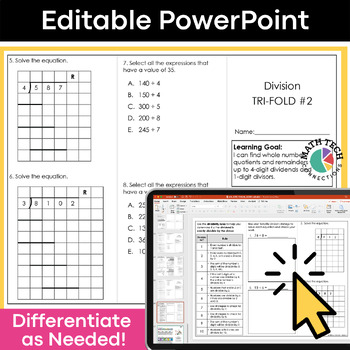Subject
Resource Type
Format
Zip (23 MB|72 pages)
Standards
\$9.00
\$9.00

#### Also included in

1. These math tri-folds are perfect for you to use during your guided math groups, math assessments, or homework. Three tri-folds per standard give you plenty material to introduce, practice, and assess all 4th grade math standards. This resource has been updated to include a Google Slides version with
\$25.00
\$42.00
Save \$17.00
2. This fourth grade math bundle includes 3 AMAZING MATH RESOURCES! Included: Math Tri-Folds, Math Sorts, and Math Morning Work!This is a MUST have resources for your 4th grade classroom. The resources included are flexible and may be used for morning work, whole group practice, guided math practice, i
\$67.50
\$118.50
Save \$51.00
3. This 4th grade math bundle includes math tri-folds that are intended for use during small group instruction and Google Forms quizzes that can be used as pre and post math assessments. This 4th grade math practice bundle includes both PRINT and DIGITAL format. - The printable format includes 84 bookl
\$40.00
\$80.00
Save \$40.00

### Description

These 4th grade math tri-folds are perfect for you to use during your guided math groups, math assessments, or homework. Three tri-folds per standard give you plenty material to introduce, practice, and assess!

This resource has been updated to include a Google Slides version with simple text boxes.

These math booklets are organized by standard, so you will save time planning your small group lessons.

STANDARDS COVERED: Number & Operations in Base 10

18 Tri-folds (3 per standard)

4.NBT.1: I can recognize that in a multi-digit whole number, a digit in one place represents ten times what it represents in the place to its right.

4.NBT.2: I can read and write multi-digit whole numbers using base-ten numerals, number names, and expanded form. I can compare two multi-digit numbers using symbols (>, <, or =) to show the comparison.

4.NBT.3: I can use what I know about place value to round multi-digit whole numbers to any place.

4.NBT.4: I can add and subtract multi-digit whole numbers using the standard algorithm.

4.NBT.5: I can multiply a whole number of up to four digits by a one-digit whole number. I can multiply two two-digit numbers.

4.NBT.6: I can find whole-number quotients and remainders with up to four-digit dividends and one-digit divisors.

* Editable Small Group Lesson Plan Template

* Editable Level of Mastery Pages

* EDITABLE WORD format of each tri-fold

Ways to use in your classroom: whole group, small group, math centers, homework, math quiz, math test, math review, math study guide

MORE MATH TRI-FOLDS

3rd Grade - Written Response Tri-Folds

4th Grade - Written Response Tri-Folds

5th Grade - Written Response Tri-Folds

Total Pages
72 pages
Included
Teaching Duration
Lifelong tool
Report this Resource to TpT
Reported resources will be reviewed by our team. Report this resource to let us know if this resource violates TpT’s content guidelines.

### Standards

to see state-specific standards (only available in the US).
Compare two decimals to hundredths by reasoning about their size. Recognize that comparisons are valid only when the two decimals refer to the same whole. Record the results of comparisons with the symbols >, =, or <, and justify the conclusions, e.g., by using a visual model.
Use decimal notation for fractions with denominators 10 or 100. For example, rewrite 0.62 as 62/100; describe a length as 0.62 meters; locate 0.62 on a number line diagram.
Express a fraction with denominator 10 as an equivalent fraction with denominator 100, and use this technique to add two fractions with respective denominators 10 and 100. For example, express 3/10 as 30/100, and add 3/10 + 4/100 = 34/100.
Solve word problems involving multiplication of a fraction by a whole number, e.g., by using visual fraction models and equations to represent the problem. For example, if each person at a party will eat 3/8 of a pound of roast beef, and there will be 5 people at the party, how many pounds of roast beef will be needed? Between what two whole numbers does your answer lie?
Understand a multiple of 𝘢/𝘣 as a multiple of 1/𝘣, and use this understanding to multiply a fraction by a whole number. For example, use a visual fraction model to express 3 × (2/5) as 6 × (1/5), recognizing this product as 6/5. (In general, 𝘯 × (𝘢/𝘣) = (𝘯 × 𝘢)/𝘣.)